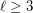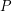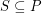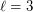# Generalised Empty Hexagon Conjecture

 Importance: Medium ✭✭
 Author(s): Wood, David R.
 Subject: Geometry
 Keywords: empty hexagon
 Posted by: David Wood on: March 26th, 2014
Conjecture   For eachthere is an integersuch that every set of at leastpoints in the plane containscollinear points or an empty hexagon.

Here an empty hexagon in a set of pointsconsists of a subsetof six points in convex position with no other point inin the convex hull of. Thecase of the conjecture (that is, for point sets in general position) was an outstanding open problem for many years, until its solution by Gerken [G] and Nicolas [N]. Valtr [V] found a simple proof.

## Bibliography

[G] Tobias Gerken. Empty Convex Hexagons in Planar Point Sets, Discrete Comput Geom (2008) 39:239–272, MathSciNet

[N] Carlos M. Nicolas. The Empty Hexagon Theorem, Discrete Comput Geom 38:389–397 (2007), MathSciNet.

[V] Pavel Valtr, On Empty Hexagons, in: J. E. Goodman, J. Pach, and R. Pollack, Surveys on Discrete and Computational Geometry, Twenty Years Later, Contemp. Math. 453, AMS, 2008, pp. 433-441.

* indicates original appearance(s) of problem.